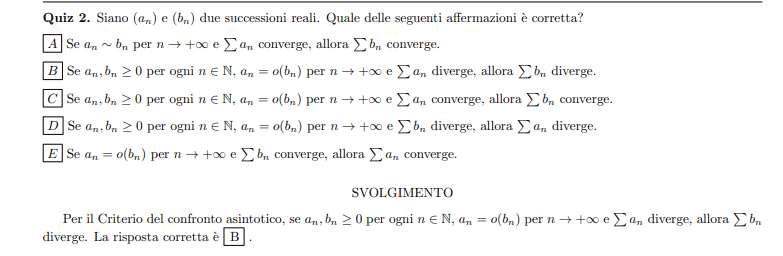# Question about the convergence of a series

• Amaelle
Statement A does not only fail where terms approaching ## n \to \infty ## may be negative: an example with strictly positive terms for both series was given in #3.f

#### Amaelle

Homework Statement
look at the image
Relevant Equations
Assymptotic approximation
Greetings!

I have a question about one assumption regarding this question even though I agree with the answer but I have a doubt about A, because
when we study the convergence of a serie we use the assymptotic approximation, so why A is not correct?
thank you!

when weExactly how is ##a_n## ~ ##b_n## defined? Is ##1/n## ~ ##1/n^2##?

•Amaelle and pbuk
Is ##1/n## ~ ##1/n^2##?

My Italian isn't great but I think in the context of the question this works better as: Does ## \frac 1 {n ^ 2} \sim \frac 1 n ## as ## n \to +\infty ##?

•FactChecker and Amaelle
My Italian isn't great but I think in the context of the question this works better as: Does ## \frac 1 {n ^ 2} \sim \frac 1 n ## as ## n \to +\infty ##?
I think they mean something like log (1+1/n) ≈ 1/n , this why I´m asking

Exactly how is ##a_n## ~ ##b_n## defined? Is ##1/n## ~ ##1/n^2##?

This is the issue.

If it means $$\lim_{n \to \infty} |a_n - b_n| \to 0$$ then indeed $1/n \sim 1/n^2$ and convergence of $\sum a_n$ tells us nothing about the convergence of $\sum b_n$.

However, to my mind $a_n \sim b_n$ as $n \to \infty$ means $$\lim_{n \to \infty} \frac{|a_n - b_n|}{|a_n|} \to 0.$$ One can show that if $a_n \sim b_n$ in this sense then there exists $N \in \mathbb{N}$ such that $$\left| \sum_{n=N}^M b_n - \sum_{n=N}^M a_n \right| < \frac12 \sum_{n=N}^M |a_n|$$ for all $M \geq N$, and it follows that if $\sum a_n$ converges absolutely then $\sum b_n$ converges absolutely. However I have not been able to show that if $\sum a_n$ converges but not absolutely then $\sum b_n$ converges, but nor have I found a counterexample.

•Amaelle
I think they mean something like log (1+1/n) ≈ 1/n , this why I´m asking
Yes I can see that. The point is that ## a_n = \frac 1 {n ^ 2}, b_n = \frac 1 n ## is a counter-example that makes statement A incorrect because we have ## a_n \sim b_n ## as ## n \to +\infty ## and we have ## \Sigma a_n ## converging to ## 2 ## but ## \Sigma b_n = \Sigma \frac 1 n ## diverges.

This all assumes an interpretation of ## a_n \sim b_n ## similar to @pasmith's first one. I read it like this:
$$a_n \sim b_n \text { as } n \to +\infty \iff \forall (\varepsilon > 0) \ \exists N : \forall (n > N) \ |a_n - b_n| < \varepsilon$$

••FactChecker and Amaelle
Yes I can see that. The point is that ## a_n = \frac 1 {n ^ 2}, b_n = \frac 1 n ## is a counter-example that makes statement A incorrect because we have ## a_n \sim b_n ## as ## n \to +\infty ## and we have ## \Sigma a_n ## converging to ## 2 ## but ## \Sigma b_n = \Sigma \frac 1 n ## diverges.

This all assumes an interpretation of ## a_n \sim b_n ## similar to @pasmith's first one. I read it like this:
$$a_n \sim b_n \text { as } n \to +\infty \iff \forall (\varepsilon > 0) \ \exists N : \forall (n > N) \ |a_n - b_n| < \varepsilon$$
amazing explanation thanks a million!

I think they mean something like log (1+1/n) ≈ 1/n , this why I´m asking
You need to determine exactly what the book with the problem means by '~'. If the book leaves it vague, then A is false. And if the book says that it means ##lim_{n\to\infty} |a_n-b_n| = 0##, A is false.

I think the problem here is that in order to use the criteria for convergence the terms of the series must be NON negative,which is not assumed in statement A. Otherwise you would have to check for the absolute convergence of the series.

•Amaelle
I think the problem here is that in order to use the criteria for convergence the terms of the series must be NON negative,which is not assumed in statement A. Otherwise you would have to check for the absolute convergence of the series.
thanks a million!

I think the problem here is that in order to use the criteria for convergence the terms of the series must be NON negative,which is not assumed in statement A. Otherwise you would have to check for the absolute convergence of the series.
Statement A does not only fail where terms approaching ## n \to \infty ## may be negative: an example with strictly positive terms for both series was given in #3.

•Amaelle
I see now, thanks a million for your feedback!